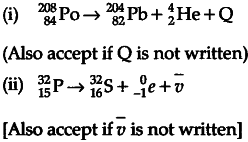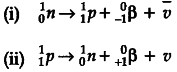# (a) Complete the following nuclear reactions

(a) Complete the following nuclear reactions:
(i)$_{ 84 }^{ 208 }Po\rightarrow \quad { 82 }^{ 204 }Pb+…$
(ii)${ 15 }^{ 32 }P\rightarrow \quad _{ 16 }^{ 32 }S+…$
(b) Write the basic process involved in nuclei responsible for (i) ${ \beta }^{ - }$ and (ii) ${ \beta }^{ + }$ decay.
© Why is it found experimentally difficult to detect
neutrinos ?

(a) We have(b) The basic processes involved are© Neutrinos are difficult to detect because :
(i) they have only weak interactions with other particles.
(ii)they can penetrate large quantity of matter
without any interaction.